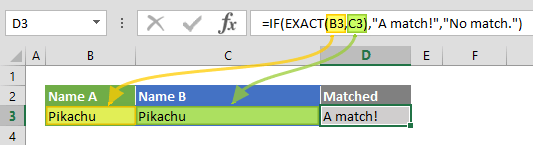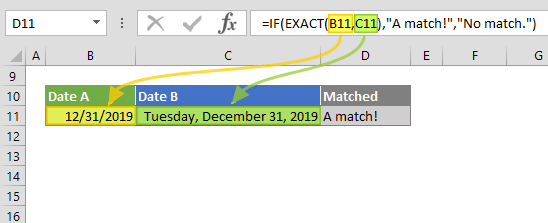Excel EXACT function compares two strings and returns TRUE for a perfect match, and FALSE if not. Note that this comparison is case-sensitive. In this guide, we’re going to show you how to use Excel EXACT function to compare strings and also go over some tips and error handling methods.

# Supported versions

• All Excel versions

# Excel EXACT Function Syntax

EXACT(text1, text2)

# Arguments

 text1 The first text string to be compared. text2 The second text string to be compared.

Examples

## Example 1

=IF(EXACT("Pikachu","Pikachu"),"A match!","No match.")
formula evaluates the EXACT function returns "A match!" text. Because, both arguments are exactly the same. As a result, comparison returns TRUE.## Example 2

=IF(EXACT("Pikachu","pikachu"),"A match!","No match.")
formula evaluates the EXACT function returns "No match." text. Because, both arguments are not exactly the same. While the first argument starts with an uppercase letter, the second doesn't. As a result, the comparison returns FALSE.# Tips

• The equal operator (=) does a similar comparison, and returns a Boolean value similar to the EXACT function. However, this comparison is not case-sensitive.
• Excel EXACT function ignores cell formatting - dates and numbers can be compared as well.